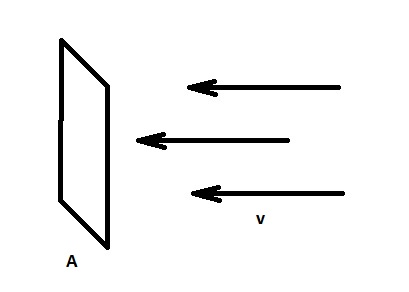# Wind energy and wind power

This online calculator computes kinetic energy of wind and wind power

Wind energy is the kinetic energy of air in motion (recall that air has density, hence, mass).

To estimate wind energy, imagine a surface with area A, which is perpendicular to the wind's direction.In this case, energy, flowing with the air with density through an imaginary surface with area with the speed during the time is

As you can see, this is the formula for kinetic energy with the mass of air expanded as the multiplication of volume and density of air.

Power is energy per unit time, so wind power is

The calculator below uses these formulae to calculate wind energy and wind power.#### Wind energy and wind power

Surface perpendicular to the direction of the wind
Default value is density of air at sea level standard pressure and temperature.
Digits after the decimal point: 2
Wind energy, Joules

Wind power, Watts

But do not forget that to use it, it should be converted into another type of energy, for example, electrical energy. As said in wikipedia, in 1919, the German physicist Albert Betz showed that for a hypothetical ideal wind-energy extraction machine, the fundamental laws of conservation of mass and energy allowed no more than 16/27 (59.3%) of the kinetic energy of the wind to be captured.

To give you an idea about wind energy and power, below are some fun facts for reference.

Energy:
The heat required to raise the temperature of 1 liter of water from 20°C to 100°C is 335 000 Joules
1 kW·h is 3 600 000 Joules
Energy of explosion of 1 tonn of TNT is 4 184 000 000 Joules

Typical power of electric kettle ranges from 800 to 2500 Watt
Eurostar train has a power of 12 000 000 Watt.

URL copied to clipboard

#### Similar calculators

PLANETCALC, Wind energy and wind power